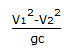# Chemical Engineering - Fluid Mechanics

21.

When the pipe Reynold's number is 6000, the flow is generally

 A. viscous B. laminar C. turbulent D. transition

Explanation:

No answer description available for this question. Let us discuss.

22.

Fanning equation is given by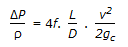. It is applicable to __________ region flow.

 A. transition B. laminar C. turbulent D. both (b) and (c)

Explanation:

No answer description available for this question. Let us discuss.

23.

With a constant diameter impeller of a centrifugal pump

 A. its capacity varies directly as the square of speed. B. head varies as the square of speed. C. horsepower input varies as the square of speed. D. head varies as the speed.

Explanation:

No answer description available for this question. Let us discuss.

24.

For water, when the pressure increases, the viscosity

 A. also increases B. decreases C. remains constant D. first decreases, and then increases

Explanation:

No answer description available for this question. Let us discuss.

25.

The head loss due to sudden expansion is

 A.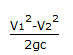B.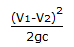C.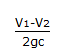D.